# ISEE Upper Level Quantitative : How to find range

## Example Questions

### Example Question #1 : Range

Examine this stem-and-leaf diagram for a set of data: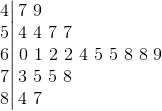Which is the greater quantity?

(a) The range of the data?

(b)(a) is greater

(a) and (b) are equal

(b) is greater

It is impossible to tell from the information given

(a) and (b) are equal

Explanation:

The "stem" of this data set represents the tens digits of the data values; the "leaves" represent the units digits.

The range of a data set is the difference of the high and low values. The highest value represented is 87 (7 is the last "leaf" in the bottom, or, 8, row); the low value is 47 (7 is the first "leaf" in the top, or, 4, row). The difference is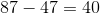, which is the range.

### Example Question #2 : Range

Consider the set of numbers: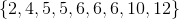Quantity A: The sum of the median and mode of the set

Quantity B: The range of the set

The two quantities are equal.

Quantity B is greater.

The relationship cannot be determined from the information given.

Quantity A is greater.

Quantity A is greater.

Explanation:

Quantity A: The median (middle number) is, and the mode (most common number) is, so the sum of the two numbers is.

Quantity B: The range is the smallest number subtracted from the largest number, which is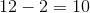.

Quantity A is larger.

### Example Question #3 : Range

In the following set of data compare the median and the range: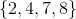The median and the range are equal

The median is greater than the range

The range is greater than the median

It is not possible to compare the mean and the mode based on the information given

The range is greater than the median

Explanation:

The median is the average of the two middle values of a set of data with an even number of values. So we have: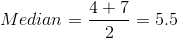The range is the difference between the lowest and the highest values. So we have: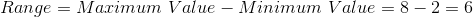So the range is greater than the median.

### Example Question #4 : Range

In the following set of data compare the mean and the range: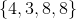The mean and the range are equal.

The range is greater than the mean.

The mean is greater than the range.

It is not possible to compare the mean and the mode based on the information given

The mean is greater than the range.

Explanation:

The mean of a set of data is given by the sum of the data, divided by the total number of values in the set. So we can write: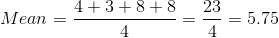The range is the difference between the lowest and the highest values. So we have: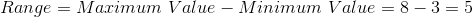So the mean is greater than the range.

### Example Question #5 : Range

In the following set of data compare the mode and the range: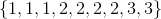The range is greater than the mode.

The mode is greater than the range.

The range is equal to the mode.

It is not possible to compare the mean and the mode based on the information given.

The range is equal to the mode.

Explanation:

The mode of a set of data is the value which occurs most frequently which isin this problem.

The range is the difference between the lowest and the highest values. So we have: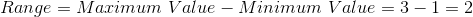So the range is equal to the mode.

### Example Question #6 : Range

Consider the following set of data: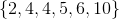Compare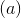and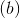.: The sum of the median and the mean of the set: The range of the set

It is not possible to compare the mean and the mode based on the information given.is greaterandare equalis greateris greater

Explanation:

The mean of a set of data is given by the sum of the data, divided by the total number of values in the set. So we can write: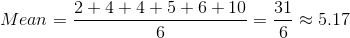The median is the average of the two middle values of a set of data with an even number of values. So we have: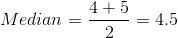So we have: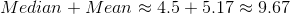The range is the difference between the lowest and the highest values. So we have: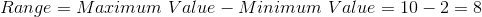Thereforeis greater than.